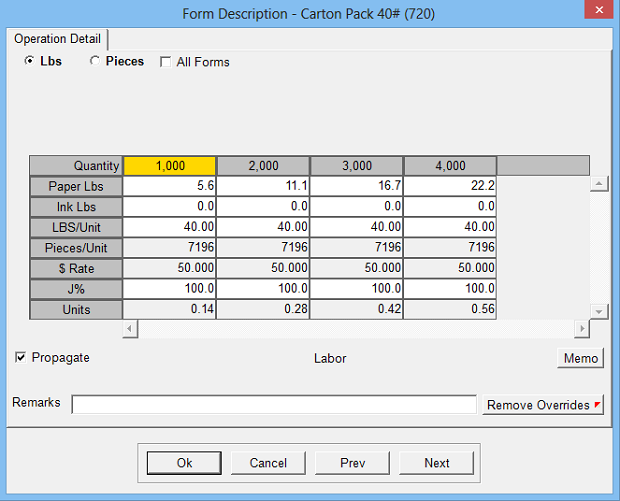Estimates the cost, time and number of units for packing operations using either pieces per unit or weight (capacity) per unit. To add the material cost of the packaging, follow this operation with a Calc 21 operation.

Operation Setup Requirements:
1. Description
2. Rate: Cost per hour
3. Sell \$ use: Markup % (recommended) or alternate sell rate
4. Calc: 20
5. Sub Key: Labor
6. Lbs per Box, Skid, etc: Capacity (pounds) per packing unit
7. Minutes First: Minutes required to pack the first unit (including setup time)
8. Minutes Repeat: Minutes required to pack each additional unit
9. Default to All Forms: Option to default to include weight of paper and ink for all formsEstimate Specification Input Prompts:

1. Lbs or Pieces: Option to override the number of calculated pieces per unit
2. All Forms: includes weight of current form or all active forms in current part
3. Paper Lbs (pounds method only)
4. Ink Lbs (pounds method only)
5. Pounds per Unit (pounds method only)
6. Pieces per Unit (pieces method only)
7. J%
8. Remarks
9. MemoCalculation:

Packing Units (pounds method) = (Paper Lbs + Ink Lbs) / Lbs per Unit

Packing Units (pieces method) = Estimate Quantity / Pieces per Unit

Minutes First + ((Packing Units - 1) x Minutes Repeat) = Standard Minutes

(Standard Minutes / 60) x J% = Estimated Hours

Estimated Hours x Rate = Cost \$

Cost \$ x Markup % = Markup \$

Cost \$ + Markup \$ = Sell \$